# Samacheer Kalvi 7th Maths Solutions Term 3 Chapter 4 Geometry Ex 4.2

Students can Download Maths Chapter 4 Geometry Ex 4.2 Questions and Answers, Notes Pdf, Samacheer Kalvi 7th Maths Book Solutions Guide Pdf helps you to revise the complete Tamilnadu State Board New Syllabus and score more marks in your examinations.

## Tamilnadu Samacheer Kalvi 7th Maths Solutions Term 3 Chapter 4 Geometry Ex 4.2

Question 1.
Draw circles for the following measurements of radius (r)/ diameters(d).
(i) r = 4 cm
(ii) d = 12 cm
(iii) r = 3.5 cm
(iv) r = 6.5 cm.
(v) d = 6 cm
Solution:
(i) r = 4 cm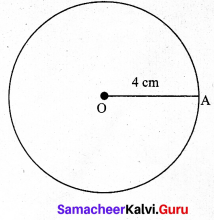Step 1 : Market a point ‘O’ on the paper.
Step 2 : Extended the compass distance equal to radius 4 cm.
Step 3 : At center ‘O’, helded the compass firmly and placed the pointed end of the compass.
Step 4 : Slowly rotated the compass around to get the circle.(ii) d = 12 cm
given d= 12 cm
∴ radius r = $$\frac { d }{ 2 }$$ = $$\frac { 12 }{ 2 }$$ = 6 cm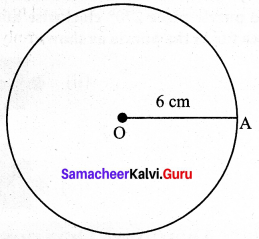Step 1: Marked a point ‘O’ on the paper.
Step 2: Extended the compass distance equal to radius 6 cm.
Step 3: At center ‘O’, held the compass firmly and placed the pointed end of the compass.
Step 4: Slowly rotated the compass around to get the circle.

(iii) r = 3.5 cm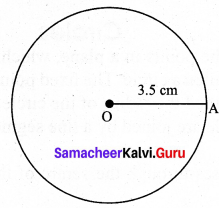Step 1: Market a point ‘O’ on the paper.
Step 2: Extended the compass distance equal to radius 3.5 cm.
Step 3: At center ‘O’, held the compass firmly and placed the pointed end of the compass.
Step 4: Slowly rotated the compass around to get the circle.(iv) r = 6.5 cm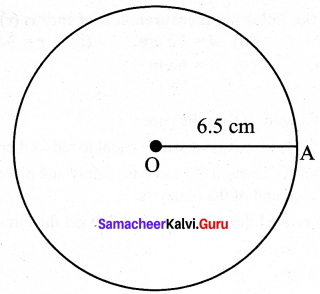Step 1: Market a point ‘O’ on the paper.
Step 2: Extended the compass distance equal to radius 6.5 cm.
Step 3: At center ‘O’, held the compass firmly and placed the pointed end of the compass.
Step 4: Slowly rotated the compass around to get the circle.

(v) d = 6 cm
∴ radius r = $$\frac { d }{ 2 }$$ = $$\frac { 6 }{ 2 }$$ = 3 cm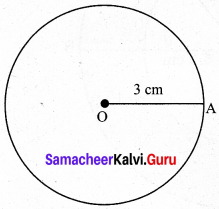Step 1: Market a point ‘O’ on the paper.
Step 2: Extended the compass distance equal to radius 3 cm.
Step 3: At center ‘O’, held the compass firmly and placed the pointed end of the compass.
Step 4: Slowly rotated the compass around to get the circle.Question 2.
Draw concentric circles for the following measurements of radii / diameters. Find out the width of each circular ring.
(i) r = 3 cm and r = 5 cm.
(ii) r = 3.5 cm and r = 6.5 cm.
(iii) d = 6.4 cm and d = 11.6 cm.
(iv) r = 5 cm and r = 7.5 cm.
(vi) r = 7.1 cm and d = 12 cm.
Solution:
(i) r = 3 cm and r = 5 cm.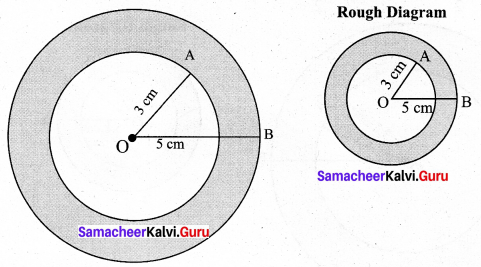Width of the circular ring = OB – OA = 5 – 3 = 2 cm
Step 1: Drawn a rough diagram and market the given measurements
Step2: Taken any point O and marked it as center.
Step 3: With O as center drawn a circle of radius OA = 3 cm.
Step 4: With O as center drawn a circle of radius OB = 5 cm. Thus concentric circles C1 and C2 are drawn.
Width of the circular ring = OB – OA = 5 – 3 = 2 cm(ii) r = 3.5 cm and r = 6.5 cm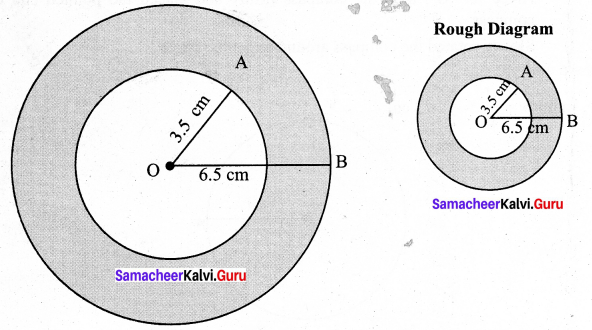Step 1: Drawn a rough diagram and market the given measurements
Step 2: Taken any point O and marked it as the center.
Step 3: With O as center drawn a circle of radius OA = 3.5 cm.
Step 4: With O as center drawn a circle of radius OB = 6.5 cm. Thus the concentric circles C1 and C2 are drawn.
Width of the circular ring = OB – OA = 6.5 – 3.5 = 3 cm(iii) d = 6.4 cm and d = 11.6 cm
r = $$\frac { d }{ 2 }$$
r = $$\frac { 6.4 }{ 2 }$$ = 3.2 cm; r = $$\frac { 11.6 }{ 2 }$$ = 5.8 cm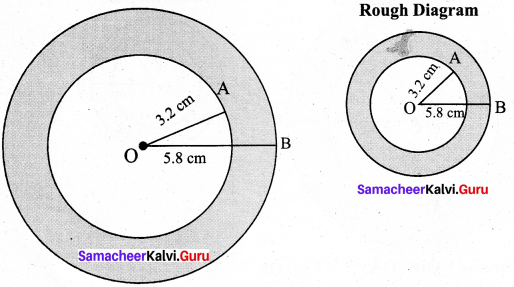Step 1: Drawn a rough diagram and market the given measurements
Step 2: Taken any point O and marked it as the center.
Step 3: With O as center drawn a circle of radius OA =3.2 cm.
Step 4: With O as center and drawn a circle of radius OB = 5.8 cm. Thus the concentric circles C1 and C2 are drawn.

Width of the circular ring = OB – OA = 5.8 – 3.2 = 2.6 cm(iv) r = 5 cm and r = 7.5 cm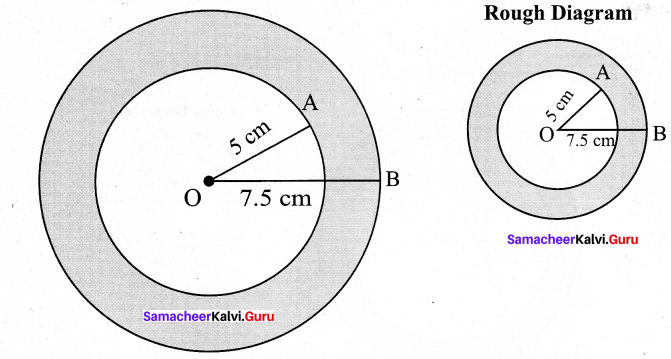Step 1 : Drawn a rough diagram and market the given measurements
Step 2 : Taken any point O and marked it as the center.
Step 3 : With O as center drawn a circle of radius OA = 5 cm.
Step 4 : With O as center drawn a circle of radius OB = 7.5 cm. Thus the concentric circles C1 and C2 are drawn.
Width of the circular ring = OB – OA = 7.5 – 5 = 2.5 cm

(v) d = 6.2 cm and r = 6.2 cm.
r = $$\frac { d }{ 2 }$$
∴ r = $$\frac { 6.2 }{ 2 }$$ = 3.1 cm and r = 6.2 cm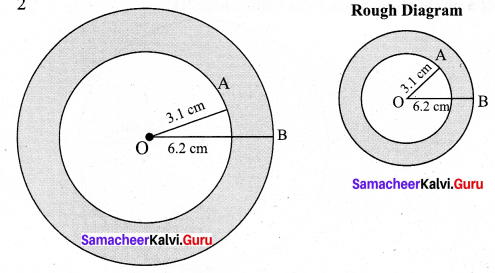Step 1: Drawn a rough diagram and market the given measurements
Step 2: Taken any point O and marked it as the center.
Step 3: With O as center, drawn a circle of radius OA = 3.1 cm.
Step 4: With O as center, drawn a circle of radius OB = 6.2 cm.
Thus the concentric circles C1 and C2 are drawn.
Width of the circular ring = OB – OA = 6.2 – 3.1 = 3.1 cm(vi) r = 7.1 cm and d = 12 cm.
r = $$\frac { d }{ 2 }$$
∴ r = $$\frac { 12 }{ 2 }$$ = 6 cm and r = 7.1 cm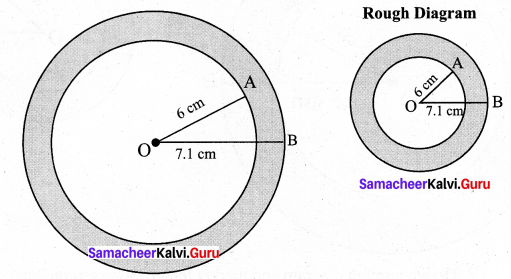Step 1: Drawn a rough diagram and marked the given measurements
Step 2 : Taken any point O and marked it as center.
Step 3 : With O as center, drawn a circle of radius OA = 6 cm.
Step 4 : with O as center, drawn a circle of radius OB = 7.1 cm. Thus concentric circles
C1 and C2 are drawn.
Width of the circular ring = OB – OA = 7.1 – 6 = 1.1 cm